Chapter 11 Prime Factors Prime Factors Prime factors

• Slides: 18Chapter 11 Prime Factors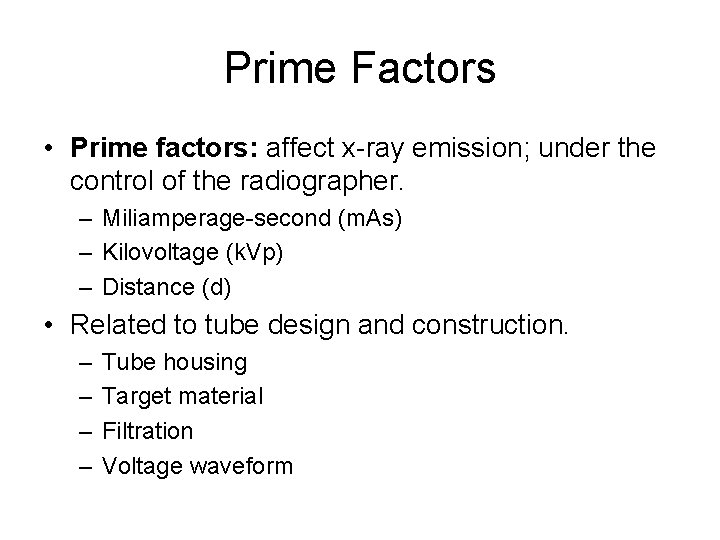Prime Factors • Prime factors: affect x-ray emission; under the control of the radiographer. – Miliamperage-second (m. As) – Kilovoltage (k. Vp) – Distance (d) • Related to tube design and construction. – – Tube housing Target material Filtration Voltage waveformX-Ray Quantity • X-ray quantity: measure of the number of x-ray photons in the useful beam. – AKA x-ray output, intensity, or exposure – Unit Roentgen (R) – Directly affected by: • Miliamperage-second (m. As) • Kilovoltage (k. Vp) • Distance (d)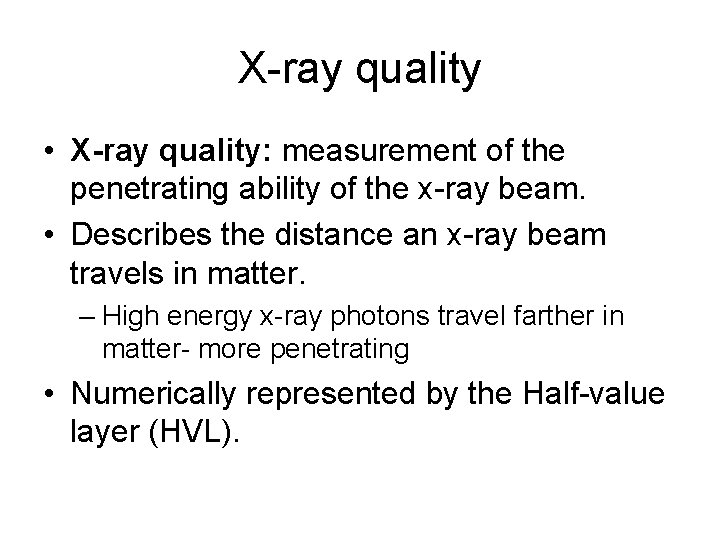X-ray quality • X-ray quality: measurement of the penetrating ability of the x-ray beam. • Describes the distance an x-ray beam travels in matter. – High energy x-ray photons travel farther in matter- more penetrating • Numerically represented by the Half-value layer (HVL).Half-Value Layer • Half-value layer of an x-ray beam is that thickness of absorbing material needed to reduce the x-ray intensity to half its original value. • Affected by: – Kilovoltage – Filtration • Not controlled from exposure to exposure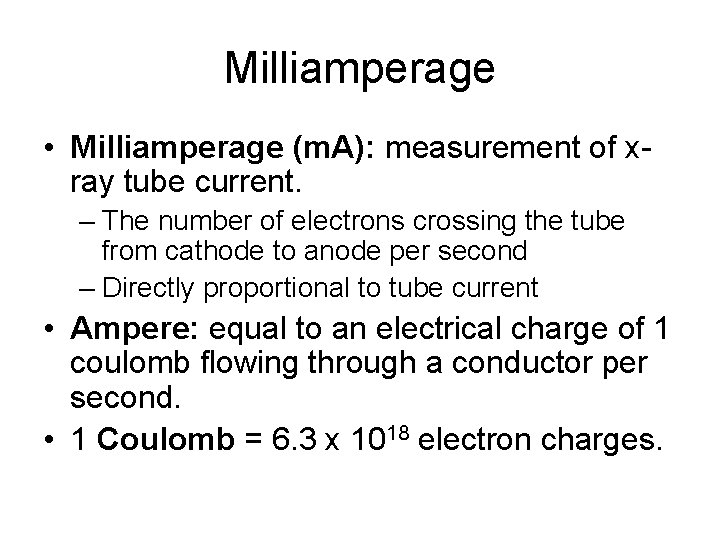Milliamperage • Milliamperage (m. A): measurement of xray tube current. – The number of electrons crossing the tube from cathode to anode per second – Directly proportional to tube current • Ampere: equal to an electrical charge of 1 coulomb flowing through a conductor per second. • 1 Coulomb = 6. 3 x 1018 electron charges.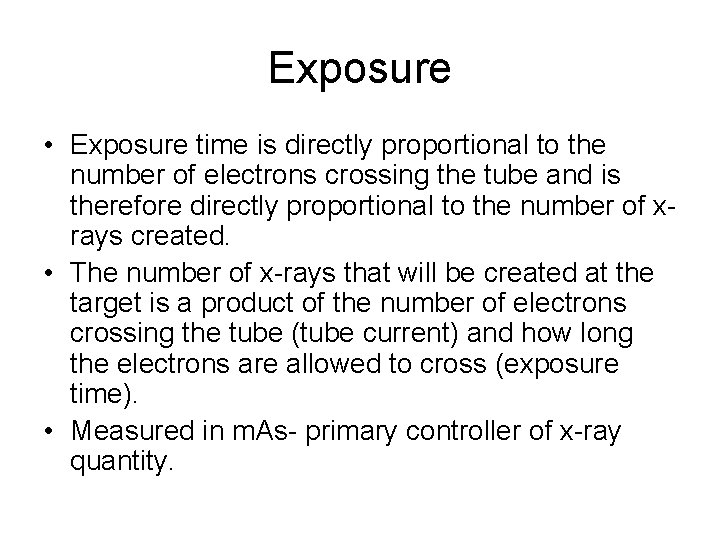Exposure • Exposure time is directly proportional to the number of electrons crossing the tube and is therefore directly proportional to the number of xrays created. • The number of x-rays that will be created at the target is a product of the number of electrons crossing the tube (tube current) and how long the electrons are allowed to cross (exposure time). • Measured in m. As- primary controller of x-ray quantity.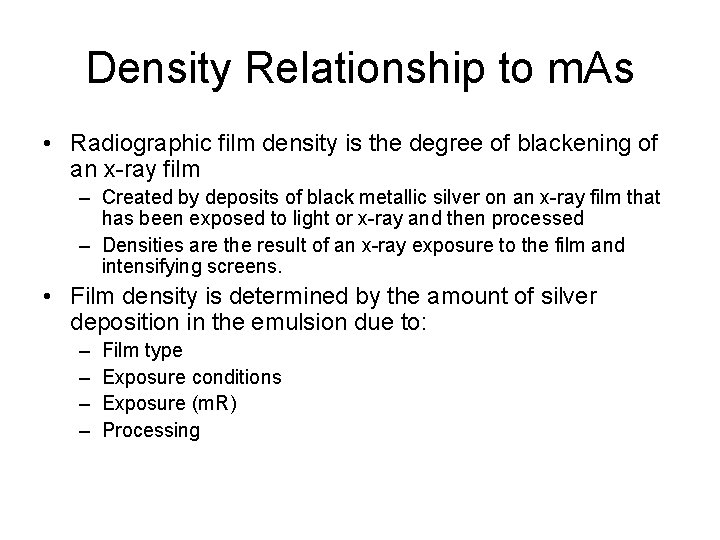Density Relationship to m. As • Radiographic film density is the degree of blackening of an x-ray film – Created by deposits of black metallic silver on an x-ray film that has been exposed to light or x-ray and then processed – Densities are the result of an x-ray exposure to the film and intensifying screens. • Film density is determined by the amount of silver deposition in the emulsion due to: – – Film type Exposure conditions Exposure (m. R) ProcessingDensity Relationship to m. As • If the exposure to a film is increased, the density to that film will increase until the point where the film reached its maximum density (Dmax). • m. As is used as the primary controller of radiographic film density. • By maintaining a specific exposure relative to the speed of the image receptor, consistent film density can be achieved.Reciprocity Law • The reaction of a photographic film to light is equal to the product of the intensity of the light and the duration of the exposure. • The density on the x-ray film should remain unchanged as long as the intensity and duration of the x-ray exposure remains unchanged. – Fails for exposures made at extremely short exposure times (less than 1/100 second) or extremely long exposure times (more than a second) • Law failure in not very significant in diagnostic radiology because exposures are seldom at those extremes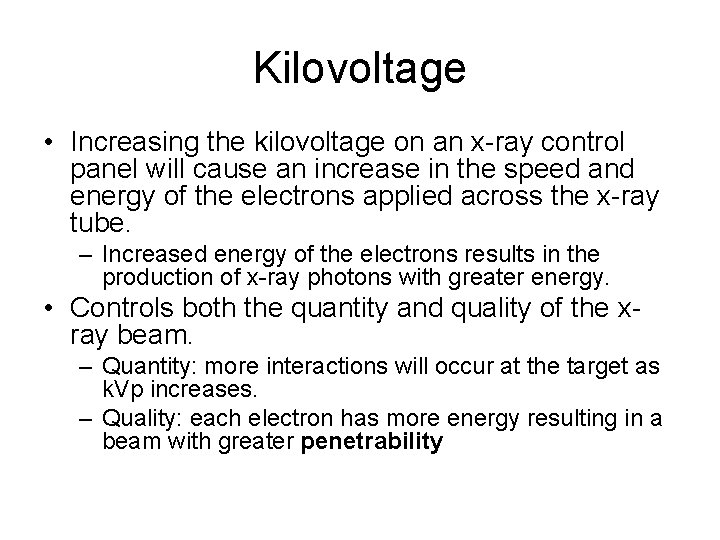Kilovoltage • Increasing the kilovoltage on an x-ray control panel will cause an increase in the speed and energy of the electrons applied across the x-ray tube. – Increased energy of the electrons results in the production of x-ray photons with greater energy. • Controls both the quantity and quality of the xray beam. – Quantity: more interactions will occur at the target as k. Vp increases. – Quality: each electron has more energy resulting in a beam with greater penetrability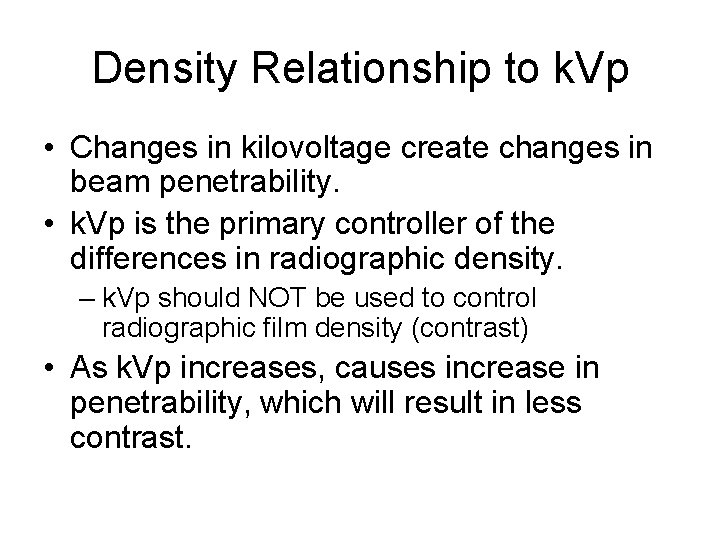Density Relationship to k. Vp • Changes in kilovoltage create changes in beam penetrability. • k. Vp is the primary controller of the differences in radiographic density. – k. Vp should NOT be used to control radiographic film density (contrast) • As k. Vp increases, causes increase in penetrability, which will result in less contrast.15 percent rule • An increase in k. Vp by 15 percent will cause a doubling in exposure, the same effect as doubling the m. A or doubling exposure time. • Hypothetically, if k. Vp is doubled, the x-ray quantity would increase by a factor of four, but this does not take into account the increased penetrability of the bean with increasing k. Vp. – As a result, radiographic density is more significantly affected. – To maintain exposure with changes in k. Vp, the 15 percent rule can be applied, – To maintain density, if the k. Vp is increased 15 percent, the m. As must be reduced to one-half its original value.Distance • The intensity of x-rays varies greatly with changes in distance. • Measurement of the x-ray intensity is obtained using a dosimeter. • X-ray photons are most concentrated at the target and from there they spread out in all directions. – X-ray intensity (quantity) begins to diminish. • Photons that exit the tube port constitute the primary useful beam.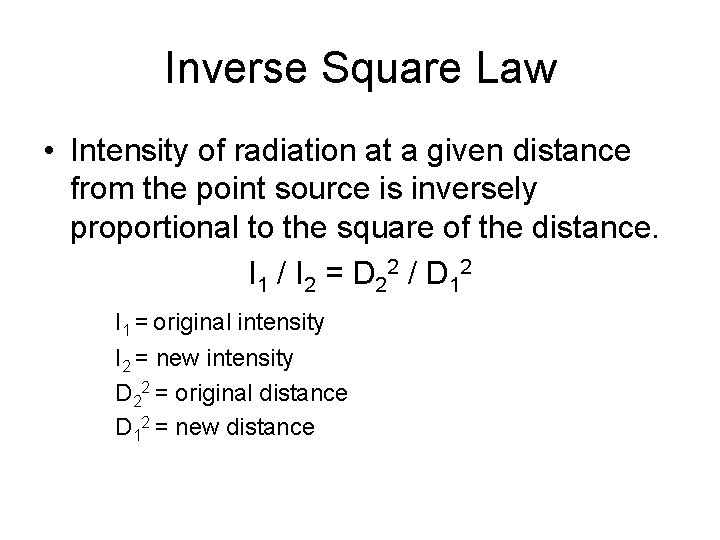Inverse Square Law • Intensity of radiation at a given distance from the point source is inversely proportional to the square of the distance. I 1 / I 2 = D 22 / D 12 I 1 = original intensity I 2 = new intensity D 22 = original distance D 12 = new distanceDistance Relationship to Distance • As the distance increases, intensity decreases which causes a decrease in exposure to the image receptor. • Since m. As is the primary controller of xray intensity and radiographic film density, m. As can be adjusted to compensate for changes in distance.Exposure (film density) maintenance formula • m. As should increase proportionally to the square of the change when distance increases. (direct square law) m. As 1 / m. As 2 = D 12 / D 22 m. As 1 = original m. As 2 = New m. As D 22 = original distance D 12 = new distanceDensity Relationship to m. As, k. Vp & d • The radiographer should select the k. Vp based on the desired contrast, and adjust m. As to provide the appropriate total exposure to the receptor.